Type
Quiz
Book Title
Fundamentals of Corporate Finance Standard Edition 9th Edition
ISBN 13
978-0073382395

### 978-0073382395 Chapter 11 Questions and Problems 23-30

April 3, 2019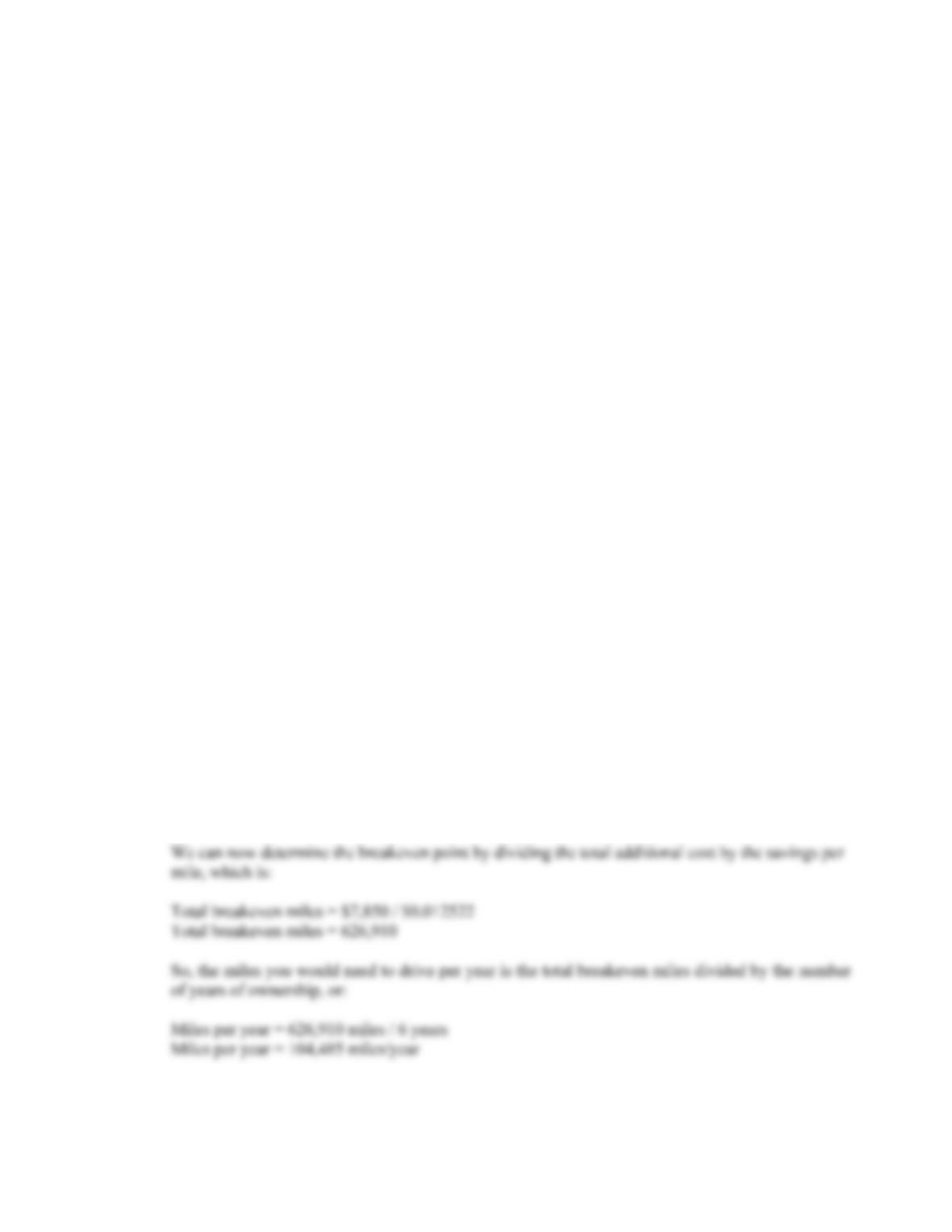CHAPTER 11 B-217
Using the bottom up OCF calculation, we get:
OCF = NI + Depreciation = \$4,005,000 + 3,200,000
OCF = \$7,205,000
The NPV at this quantity is:
NPV = –\$22,400,000 – \$1,250,000 + \$7,205,000(PVIFA10%,7) + \$1,250,000/1.107
NPV = \$12,068,405.23
So, the sensitivity of the NPV to changes in the quantity sold is:
NPV/Q = (\$10,841,563.69 – 12,068,405.23)/(51,000 – 52,000)
NPV/Q = \$1,226.84
For an increase (decrease) of one set of clubs sold per year, the NPV increases (decreases) by \$1,226.84.
23. a. First we need to determine the total additional cost of the hybrid. The hybrid costs more to
purchase and more each year, so the total additional cost is:
Total additional cost = \$5,450 + 6(\$400)
Next, we need to determine the cost per mile for each vehicle. The cost per mile is the cost per
gallon of gasoline divided by the miles per gallon, or:
Cost per mile for traditional = \$3.60/23
Cost per mile for traditional = \$0.156522
Cost per mile for hybrid = \$3.60/25
Cost per mile for hybrid = \$0.144000
So, the savings per mile driven for the hybrid will be:
Savings per mile = \$0.156522 – 0.144000
Savings per mile = \$0.012522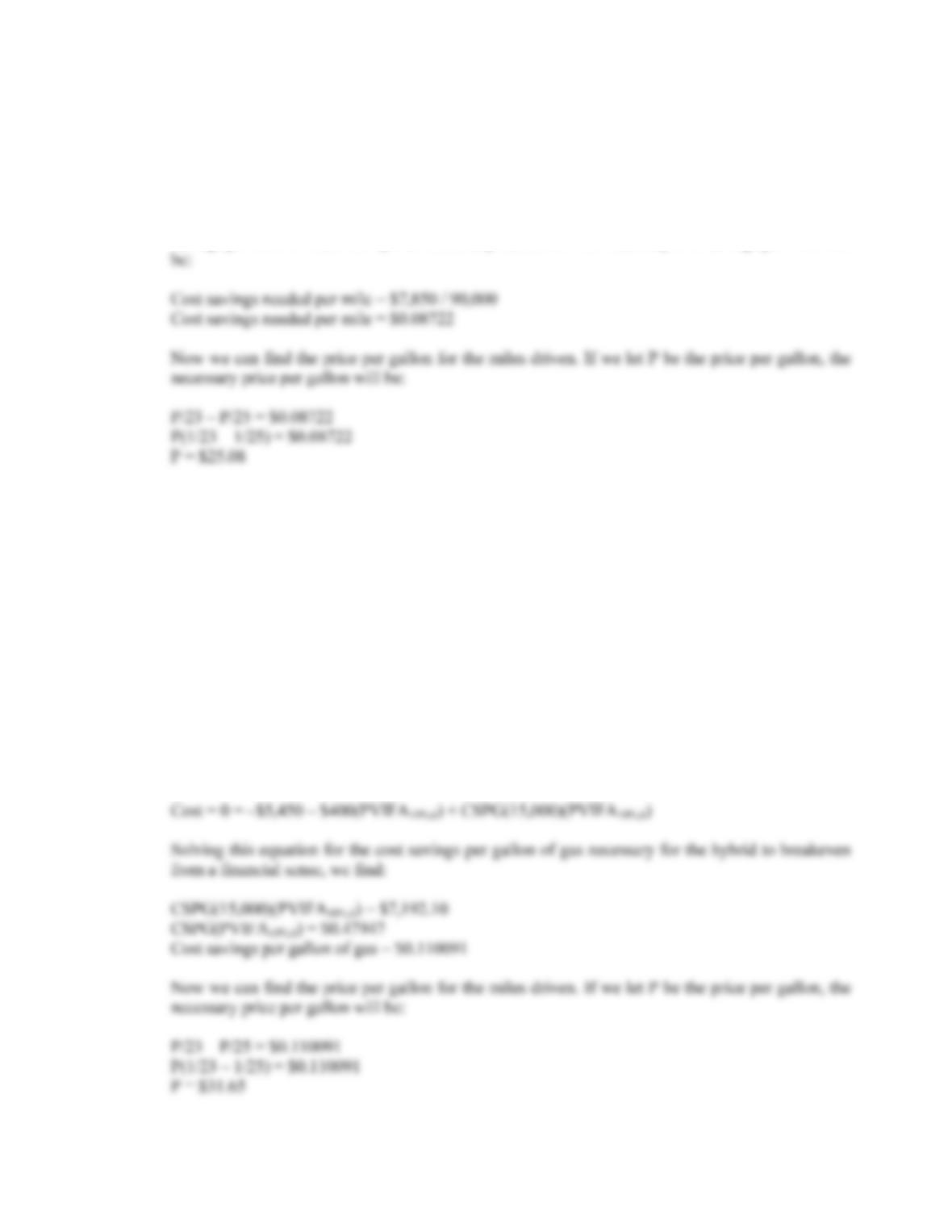B-218 SOLUTIONS
b. First, we need to determine the total miles driven over the life of either vehicle, which will be:
Total miles driven = 6(15,000)
Total miles driven = 90,000
Since we know the total additional cost of the hybrid from part a, we can determine the necessary
savings per mile to make the hybrid financially attractive. The necessary cost savings per mile will
c. To find the number of miles it is necessary to drive, we need the present value of the costs and
savings to be equal to zero. If we let MDPY equal the miles driven per year, the breakeven
equation for the hybrid car as:
Cost = 0 = –\$5,450 – \$400(PVIFA10%,6) + \$0.012522(MDPY)(PVIFA10%,6)
The savings per mile driven, \$0.012522, is the same as we calculated in part a. Solving this
equation for the number of miles driven per year, we find:
\$0.012522(MDPY)(PVIFA10%,6) = \$7,192.10
MDPY(PVIFA10%,6) = 574,369.44
Miles driven per year = 131,879
To find the cost per gallon of gasoline necessary to make the hybrid break even in a financial
sense, if we let CSPG equal the cost savings per gallon of gas, the cost equation is: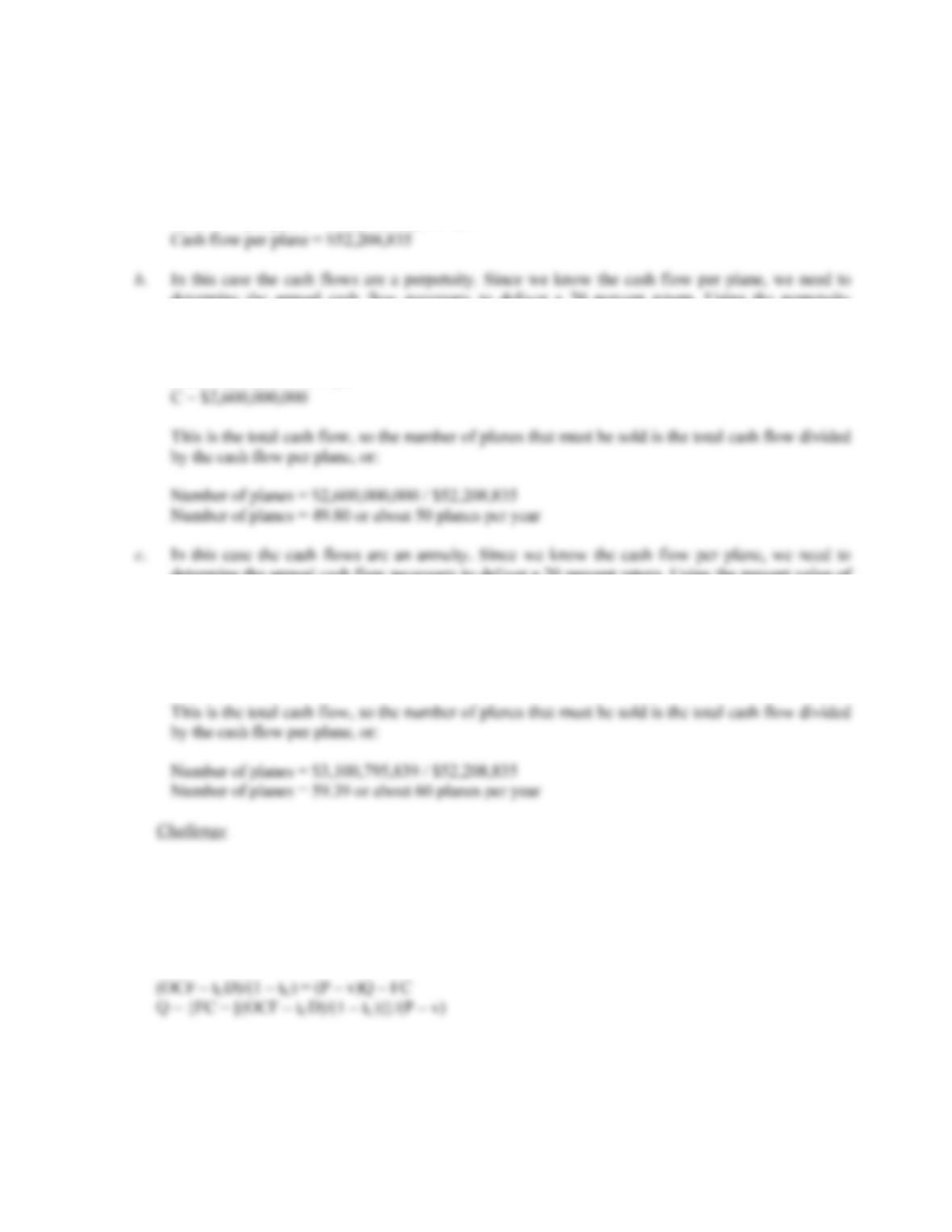CHAPTER 11 B-219
d. The implicit assumption in the previous analysis is that each car depreciates by the same dollar
amount.
24. a. The cash flow per plane is the initial cost divided by the breakeven number of planes, or:
Cash flow per plane = \$13,000,000,000 / 249
determine the annual cash flow necessary to deliver a 20 percent return. Using the perpetuity
equation, we find:
PV = C /R
\$13,000,000,000 = C / .20
determine the annual cash flow necessary to deliver a 20 percent return. Using the present value of
an annuity equation, we find:
PV = C(PVIFA20%,10)
\$13,000,000,000 = C(PVIFA20%,10)
C = \$3,100,795,839
25. a. The tax shield definition of OCF is:
OCF = [(P – v)Q – FC ](1 – tC) + tCD
Rearranging and solving for Q, we find: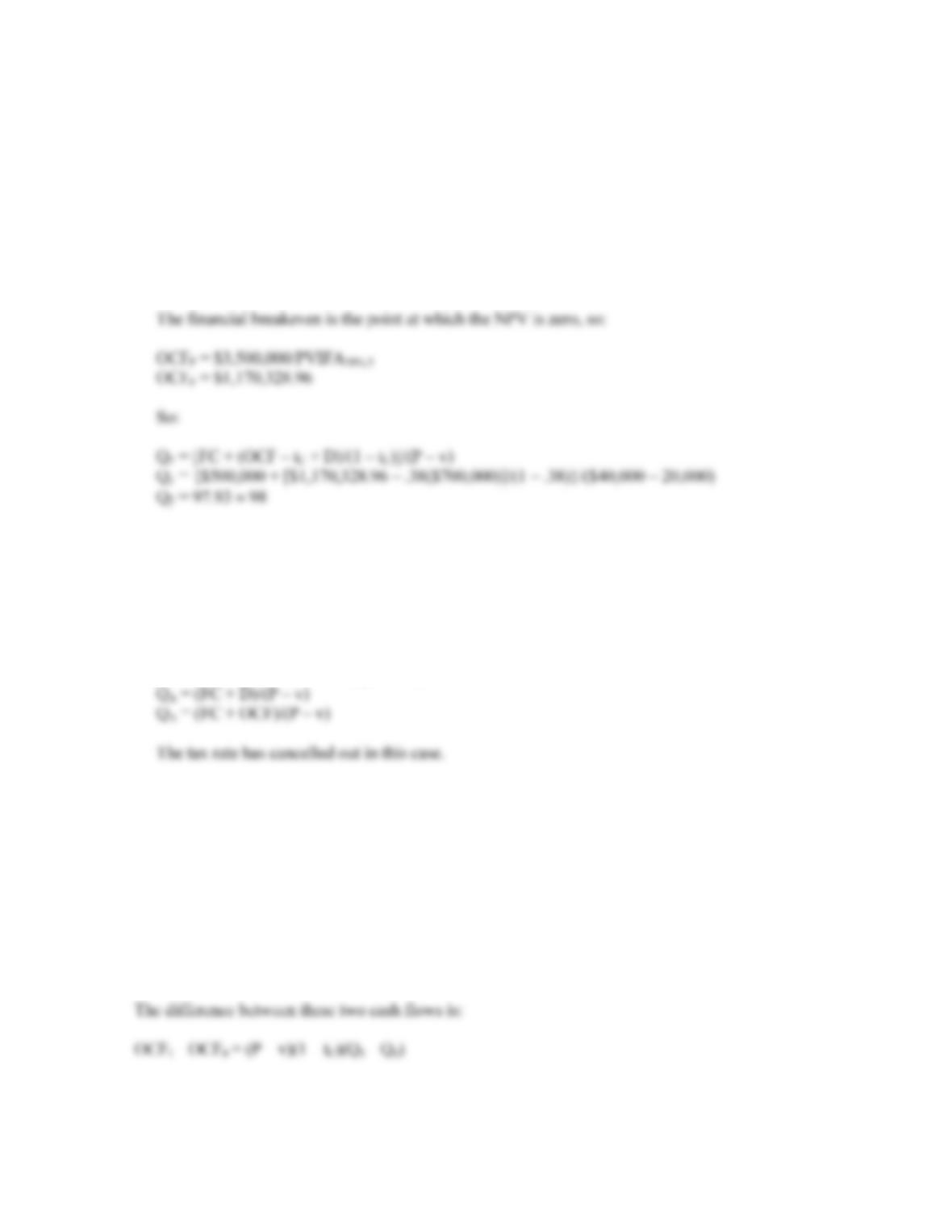B-220 SOLUTIONS
b. The cash breakeven is:
Q
C = \$500,000/(\$40,000 – 20,000)
Q
C = 25
And the accounting breakeven is:
Q
A = {\$500,000 + [(\$700,000 – \$700,000(0.38))/0.62]}/(\$40,000 – 20,000)
Q
A = 60
c. At the accounting break-even point, the net income is zero. This using the bottom up definition of
OCF:
OCF = NI + D
We can see that OCF must be equal to depreciation. So, the accounting breakeven is:
Q
A = {FC + [(D – tCD)/(1 – t)]}/(P – v)
Q
26. The DOL is expressed as:
DOL = %OCF / %Q
DOL = {[(OCF1 – OCF0)/OCF0] / [(Q1 – Q0)/Q0]}
The OCF for the initial period and the first period is:
OCF1 = [(P – v)Q1 – FC](1 – tC) + tCD
OCF0 = [(P – v)Q0 – FC](1 – tC) + tCD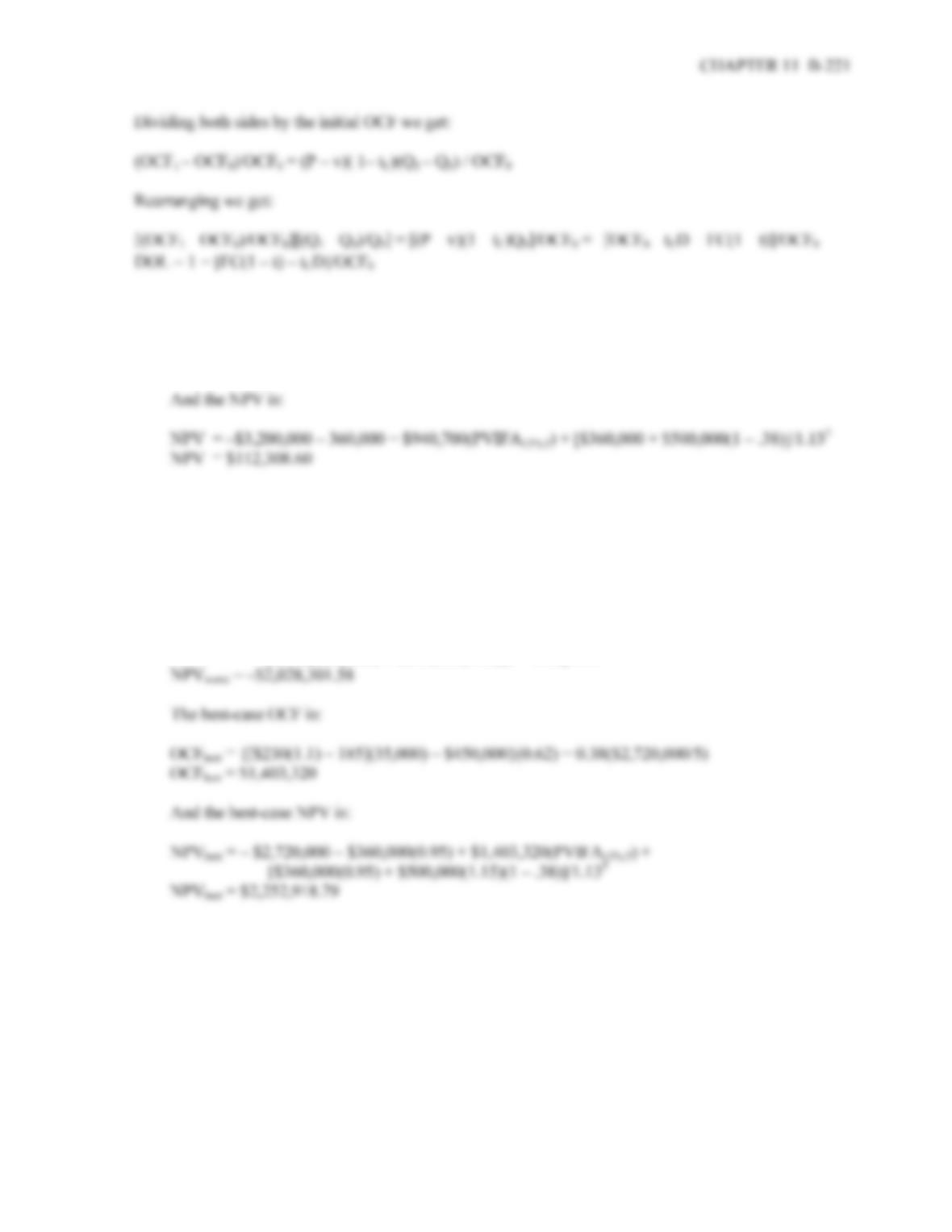27. a. Using the tax shield approach, the OCF is:
OCF = [(\$230 – 185)(35,000) – \$450,000](0.62) + 0.38(\$3,200,000/5)
OCF = \$940,700
b. In the worst-case, the OCF is:
OCFworst = {[(\$230)(0.9) – 185](35,000) – \$450,000}(0.62) + 0.38(\$3,680,000/5)
OCFworst = \$478,080
And the worst-case NPV is:
NPVworst = –\$3,680,000 – \$360,000(1.05) + \$478,080(PVIFA13%,5) +
[\$360,000(1.05) + \$500,000(0.85)(1 – .38)]/1.135
28. To calculate the sensitivity to changes in quantity sold, we will choose a quantity of 36,000. The OCF at
this level of sale is:
OCF = [(\$230 – 185)(36,000) – \$450,000](0.62) + 0.38(\$3,200,000/5)
OCF = \$968,600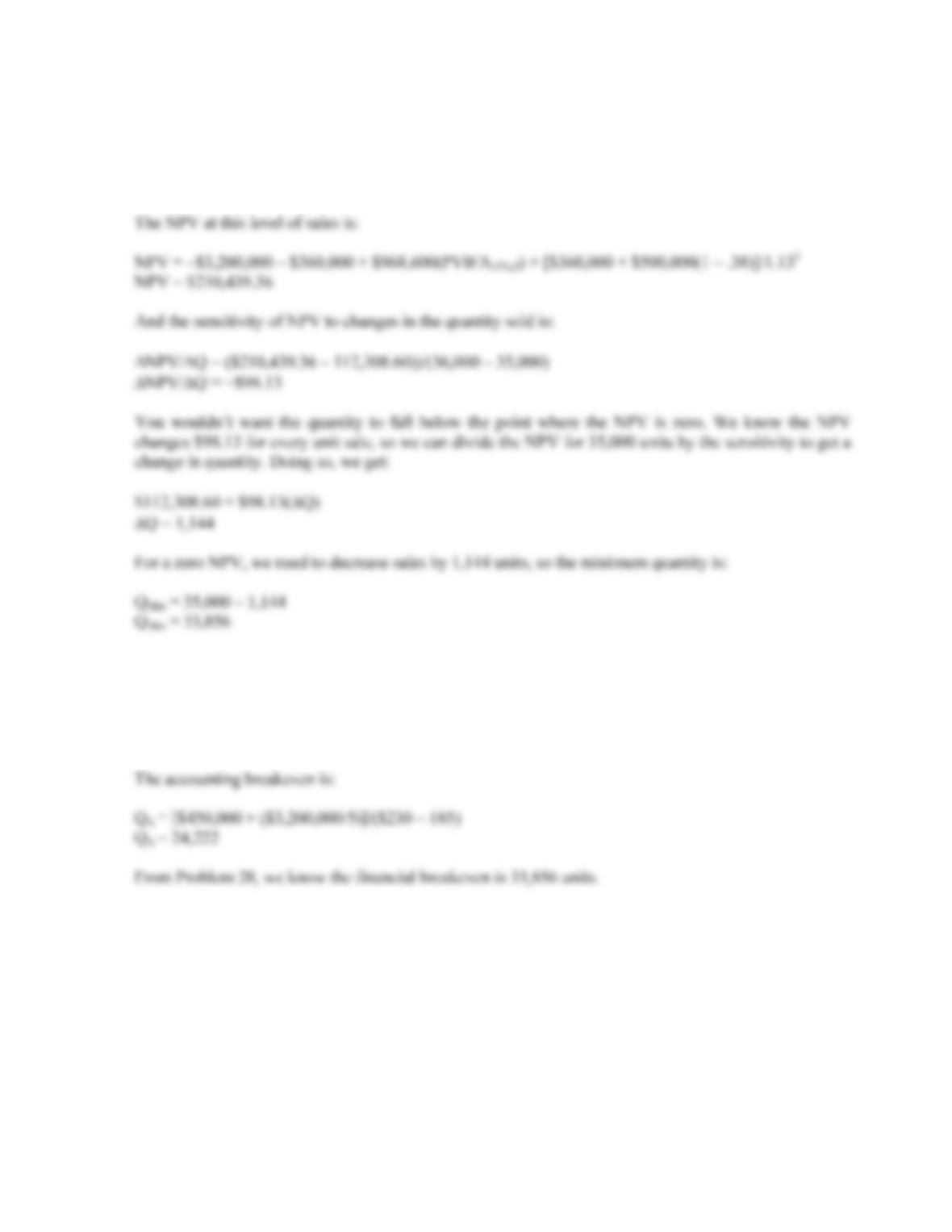B-222 SOLUTIONS
The sensitivity of changes in the OCF to quantity sold is:
OCF/Q = (\$968,600 – 940,700)/(36,000 – 35,000)
OCF/Q = +\$27.90
29. At the cash breakeven, the OCF is zero. Setting the tax shield equation equal to zero and solving for the
quantity, we get:
OCF = 0 = [(\$230 – 185)QC – \$450,000](0.62) + 0.38(\$3,200,000/5)
Q
C = 1,283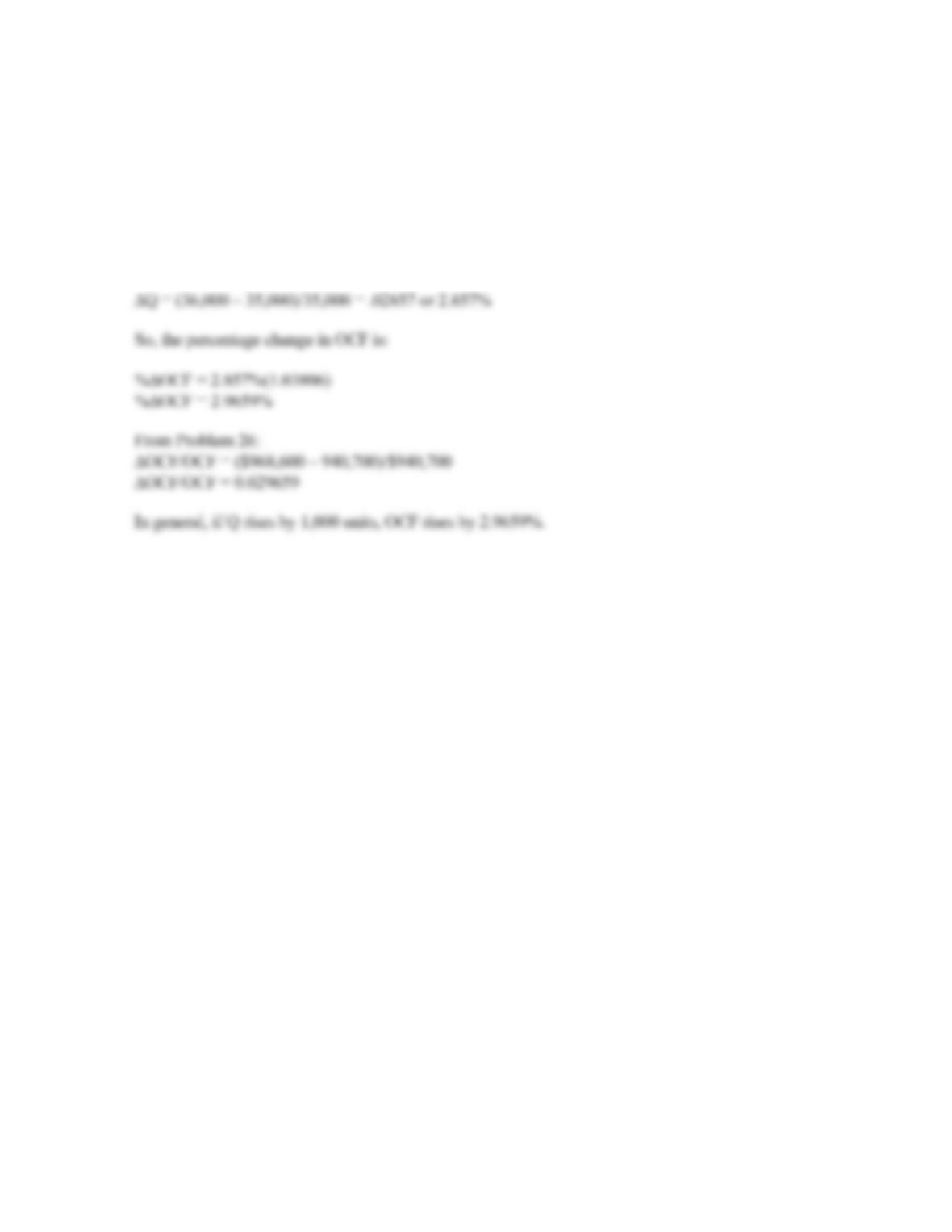CHAPTER 11 B-223
30. Using the tax shield approach to calculate the OCF, the DOL is:
DOL = 1 + [\$450,000(1 – 0.38) – 0.38(\$3,200,000/5)]/ \$940,700
DOL = 1.03806
Thus a 1% rise leads to a 1.03806% rise in OCF. If Q rises to 36,000, then
The percentage change in quantity is: